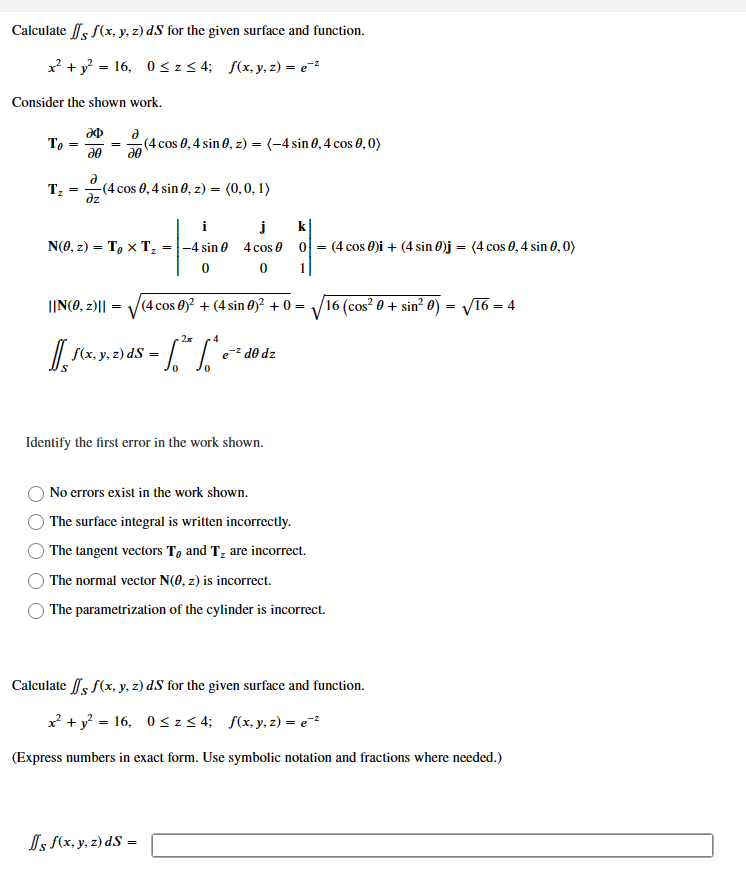Home / Expert Answers / Calculus / calculate-sf-x-y-z-ds-for-the-given-surface-and-function-x2-y2-16-0z4-f-x-y-z-ez-c-pa850

# (Solved): Calculate Sf(x,y,z)dS for the given surface and function. x2+y2=16,0z4;f(x,y,z)=ez C ...Calculate for the given surface and function. Consider the shown work. Identify the first error in the work shown. No errors exist in the work shown. The surface integral is written incorrectly. The tangent vectors and are incorrect. The normal vector is incorrect. The parametrization of the cylinder is incorrect. Calculate for the given surface and function. (Express numbers in exact form. Use symbolic notation and fractions where needed.)

We have an Answer from Expert